# 二叉树的公共最近祖先问题

## 二叉搜索树的公共最近祖先问题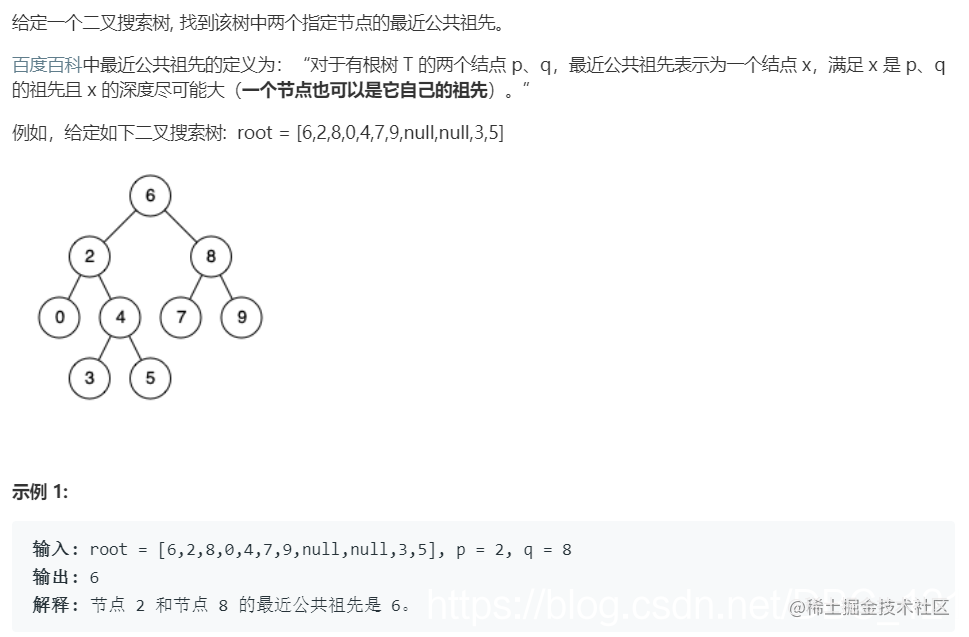• 节点 N 左子树上的所有节点的值都小于等于节点 N 的值
• 节点 N 右子树上的所有节点的值都大于等于节点 N 的值
• 左子树和右子树也都是 二叉搜索树(BST)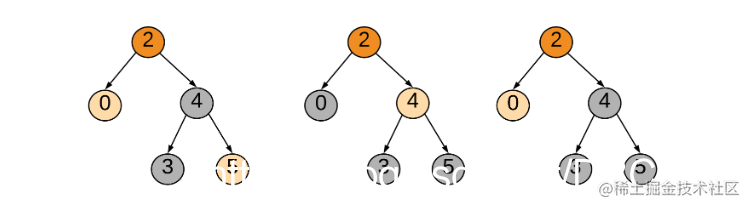• 从根节点开始遍历树
• 如果节点 p 和节点 q 都在右子树上，那么以右孩子为根节点继续 1 的操作
• 如果节点 p 和节点 q 都在左子树上，那么以左孩子为根节点继续 1 的操作
• 如果条件 2 和条件 3 都不成立，这就意味着我们已经找到节 p 和节点 q 的 LCA 了

``````public TreeNode lowestCommonAncestor(TreeNode root, TreeNode p, TreeNode q) {
if (p.val < root.val && q.val < root.val) {
return lowestCommonAncestor(root.left, p, q);
}else if (p.val > root.val && q.val > root.val) {
return lowestCommonAncestor(root.right, p, q);
}else return root;
}

## 二叉树的公共最近祖先问题

• 从根节点开始遍历树。
• 通过循环迭代，在找到 p 和 q 之前，将父指针存储在字典中。
• 一旦我们找到了 p 和 q，我们就可以使用父指针字典获得 p 的所有祖先，并添加到一个称为祖先的集合中。
• 同样，我们遍历节点 q 的祖先。如果祖先存在于为 p 设置的祖先中，这意味着这是 p 和 q 之间的第一个公共祖先（同时向上遍历），因此这是 LCA 节点。
``````public TreeNode lowestCommonAncestor(TreeNode root, TreeNode p, TreeNode q) {
Deque<TreeNode> stack = new ArrayDeque<>();
Map<TreeNode, TreeNode> parent = new HashMap<>();

parent.put(root, null);
stack.push(root);

//通过一层一层的遍历，知道遍历到两个节点为止
while (!parent.containsKey(p) || !parent.containsKey(q)) {
TreeNode node = stack.pop();

if (node.left != null) {
parent.put(node.left, node);
stack.push(node.left);
}
if (node.right != null) {
parent.put(node.right, node);
stack.push(node.right);
}
}

Set<TreeNode> ancestors = new HashSet<>();

while (p != null) {
p = parent.get(p);
}

while (!ancestors.contains(q))
q = parent.get(q);
return q;
}

#### 不记录路径思路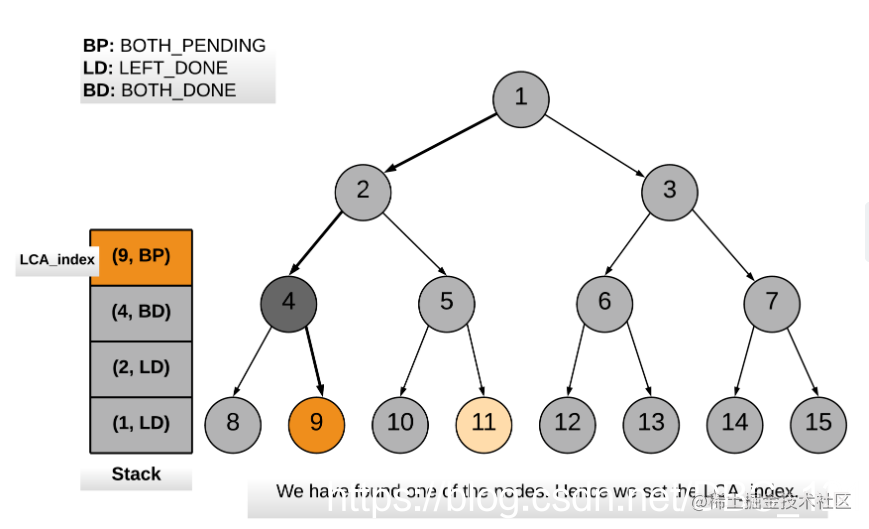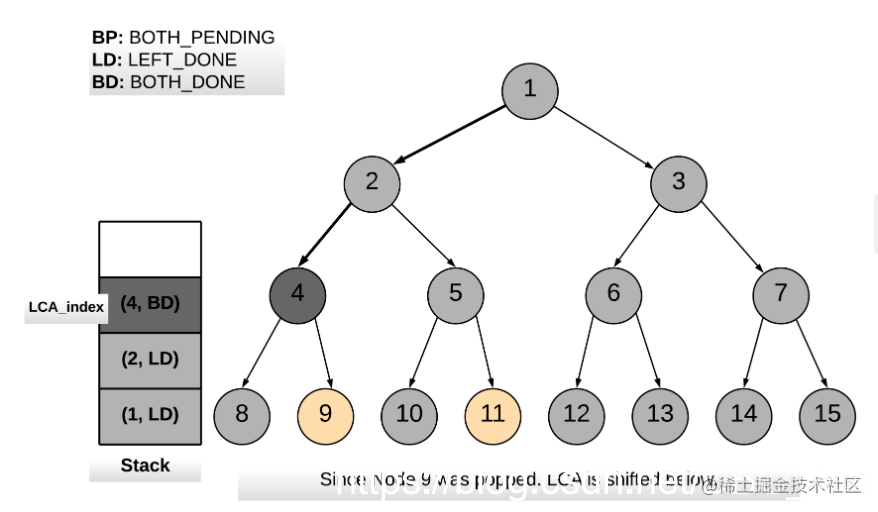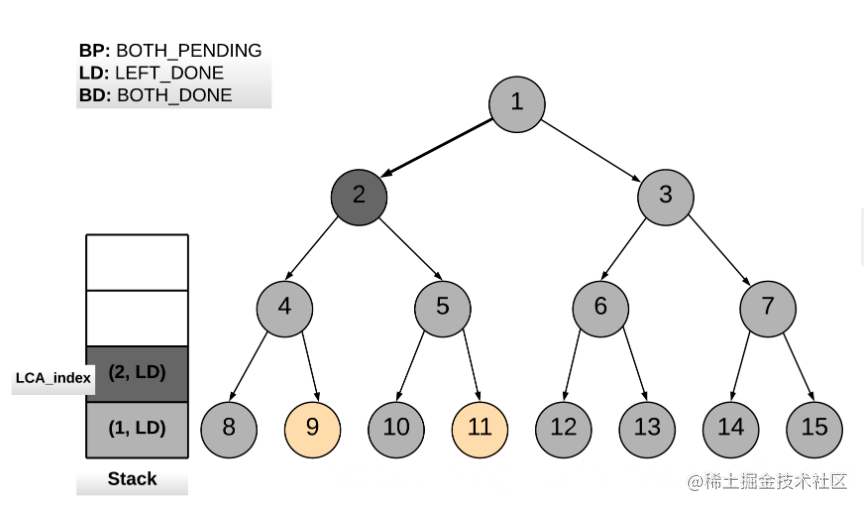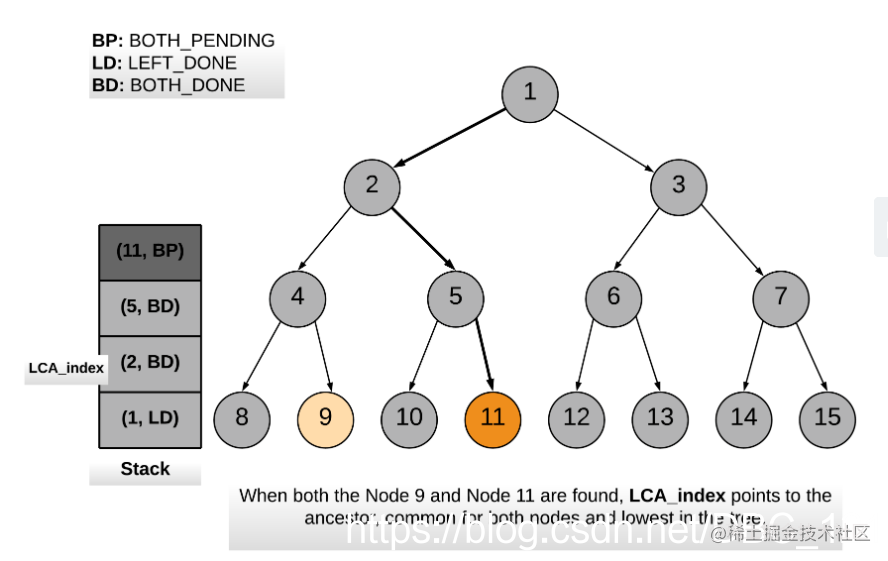``````private static int BOTH_PENDING = 2;
private static int LEFT_DONE = 1;
private static int BOTH_DONE = 0;

public TreeNode lowestCommonAncestor(TreeNode root, TreeNode p, TreeNode q) {

Stack<Pair<TreeNode, Integer>> stack = new Stack<Pair<TreeNode, Integer>>();
stack.push(new Pair<TreeNode, Integer>(root, Solution.BOTH_PENDING));

boolean one_node_found = false;
TreeNode LCA = null;
TreeNode child_node = null;

while (!stack.isEmpty()) {

Pair<TreeNode, Integer> top = stack.peek();
TreeNode parent_node = top.getKey();
int parent_state = top.getValue();

if (parent_state != Solution.BOTH_DONE) {
if (parent_state == Solution.BOTH_PENDING) {
if (parent_node == p || parent_node == q) {
if (one_node_found) {
return LCA;
} else {
one_node_found = true;
LCA = stack.peek().getKey();
}
}
child_node = parent_node.left;
} else {
child_node = parent_node.right;
}
stack.pop();
stack.push(new Pair<TreeNode, Integer>(parent_node, parent_state - 1));

if (child_node != null) {
stack.push(new Pair<TreeNode, Integer>(child_node, Solution.BOTH_PENDING));
}
} else {
if (LCA == stack.pop().getKey() && one_node_found) {
LCA = stack.peek().getKey();
}
}
}
return null;
}

#### 递归思路

• 从根节点开始遍历树。
• 如果当前节点本身是 p 或 q 中的一个，我们会将变量 mid 标记为 true，并继续搜索左右分支中的另一个节点。
• 如果左分支或右分支中的任何一个返回 true，则表示在下面找到了两个节点中的一个。
• 如果在遍历的任何点上，left、right 或者 mid 三个标记中的任意两个变为 true，这意味着我们找到了节点 p 和 q 的最近公共祖先。
``````private TreeNode res;
public boolean recurseTree(TreeNode currentNode, TreeNode p, TreeNode q) {
if (currentNode == null) return false;

int left = recurseTree(currentNode.left, p, q) ? 1 : 0;
int right = recurseTree(currentNode.right, p, q) ? 1 : 0;

int mid = 0;
if (currentNode.val == p.val || currentNode.val == q.val) mid = 1;

if (left + right + mid >= 2) {
res = currentNode;
return false;
} else if (left + right + mid > 0) {
return true;
} else return false;
}

public TreeNode lowestCommonAncestorS1(TreeNode root, TreeNode p, TreeNode q) {
recurseTree(root, p, q);
return res;
}